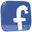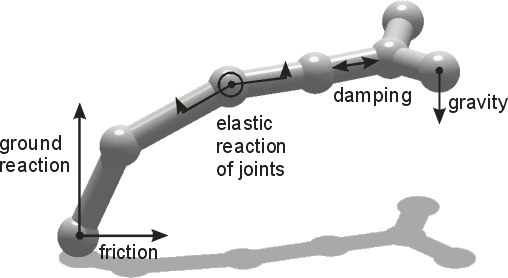Body simulation

Bodies of creatures are divided into small pieces (at sticks' ends) which are ideal material points. This approach is called "finite element method": not every point of a material body is simulated – only a finite number of points, representing small volumes in the body. The simulator calculates all the forces affecting a given point: gravity, elastic reaction when joined with other points, ground reaction and friction when touching the ground, etc.

In our model some assumptions are taken to simplify calculations. A primitive but fast numeric integration method is used, so the results are not very exact when dealing with big forces.

 The picture on the right shows sample forces calculated in the Framsticks physical simulator, Mechastick. The body is made from parts (points) and joints (sticks, rods).Another simulation engine that can be used within Framsticks is ODE.